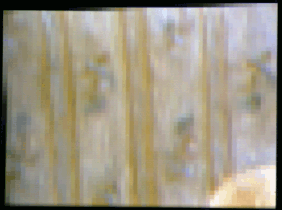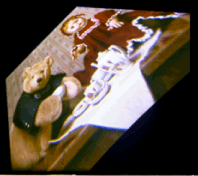Theseus Research : Technical Papers: A Nonaliasing, Real-Time Spatial Transform Technique Page 6 of 6

Summary

The four target corner points are determined by applying the traditional coordinate transform equations. The remainder of the transform is accomplished by providing output location and size factor values to the interpolation algorithm for each column of the first pass and each row of the second pass.

Because the interpolation from the input image is complete, contiguous, and continuous, there are no resampling artifacts in the interior of the output image. Because each row and each column is mapped with subpixel position phasing, there is no edge aliasing of the output image. The output image is generated directly in the form that anti-aliasing attempts to achieve under conventional approaches to spatial image transform. Furthermore, the transformation is achieved with relatively simple computations.

Figure 13, Figure 14 and Figure 15 illustrate a 30-degree rotation. Figure 16 is a 2-degree rotation and shrink by 1/2. Figure 17 shows the upper-left corner of the 2-degree rotation magnified by pixel replication to show the partial-intensity edge pixels which avoid edge aliasing. A true-perspective mapping and various arbitrary mappings have also been developed within the technique. Figure 18 and Figure 19 show the intermediate an final images of a true-perspective mapping. Figure 20 shows a mapping of the image driven by a sine wave.

The following images have been through much abuse and are included here only to provide continuity with the original article. Please do not take these images as indicative of the quality of the algorithm as quality may vary with monitor and printer.

The images in Figures 13 through 20 are reproduced with the permission of Jean Grapp.

 Figure 13. The original image.Figure 14. Thirty-degree rotation; intermediate image.Figure 15. Thirty-degree rotation; final image.Figure 16. Two-degree rotation and shrink by 1/2.Figure 17. Partial-intensity edges of the 2-degree rotation.Figure 18. True-perspective mapping; intermediate image.Figure 19. True-respective mapping; final image.Figure 20. Sine-wave mapping in both directions.Conclusion

The one-dimensional resampling interpolation technique is computationally simple, deterministically bounded, and provides a high-fidelity mapping from discrete input pixels to discrete output pixels. It can be combined in two passes over a two-dimensional image to effect two-dimensional spatial transforms. Real-time systems using the technique can be implemented more economically and perform higher fidelity transforms than systems using the standard technique of mapping points first and then interpolating intensities.

References

 1 E. Catmul and A.R. Smith, "3D Transformations of Images in Scanline Order, Computer Graphics (Proc. SIGGRAPH 80), Vol. 14, No. 3, July 1980, pp.279-286. 2 M. Shantz, "Two Pass Warp Algorithm for Hardware Implementation," Proc. SPIE. Vol. 306, "Processing and Display of Three Dimensional Data," 1982, pp.l60-164.

Footnotes
1.This is a rerendering of: Karl Fant, "A Nonaliasing, Real-Time Spatial Transform Technique", IEEE Computer Graphics and Applications, January 1986, pages 71-80.